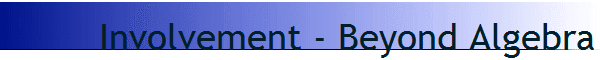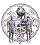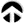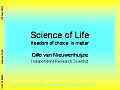### Beyond Operator Algebra

Most people know, and regard, mathematics as a method for calculating ‘values’.
Students of mathematics learn that mathematics serves a more general function.
Advanced students of mathematics learn about mathematical transform, and (sometimes) about transforming mathematics, by changing mathematical operators.
In this quest, of the mathematics of involvement, we need to go beyond operator algebra; we need to understand algebraic equations - we need to understand that/how the equation sign represents us.

### Mathematics

Mathematics is a languaging in which it is possible to define the objects of observation in an abstract manner,
to describe the relationships between them in an explicit formal sense,
to address the transformations involved in an explicit implied manner,
and to deal with the outcome/meaning of the observation by means of the equation as representation of the dynamics of observation.

In the mathematical equations we can see that there are usually four elements in the description:

1. First there are the “parameters” which can be fixed or changed (variable) depending on the specific use of the mathematician.
2. then there are the “variables” or ‘the unknowns’ which are explicitly specified by the mathematician.
3. then there are the “operators” for the mathematical relationships/transformations witch are both implicitly and explicitly defined.
4. and finally there is the “equation signwhich actually represents the position of the Observer in the observation.

### MatheMagics

In my paper “MatheMagics” I addressed this role of the equation sign as the implied representative of the mathematician.

I there showed a graphic dynamic image of the journey of the equation sign during the steps of the mathematical operation.
it was shown as the moving of the equation sign within the field of observation, up to its boundary and beyond, reflecting and reverberating in the fields of observation which has multiple potentials and finally simplifying to the equation sign centred in the field of observation.
Thereby it is possible to see how the equation sign can go through various observations of linear, variational, matrix multiplicities, and transcendental observations.

It is essential to understand that the equation sign in mathematics has no reality.
As every description in science it is linguistic model.
In fact the whole reality of ‘models’ is a linguistic reality only.
So-called objects in science are linguistic object only.

### Language

Mathematics is a language in which it is possible to define, and redefine, such linguistic models.
Contrary to spoken language, mathematics has the ability to transform and re-form the descriptions that are used.
As a result, it is possible to change the mode of observation during the observation itself.
That is why mathematics can be difficult to understand for beginners; because of its explicit use of abstraction.

The 1st operation in mathematics is always that of dis-identification: no longer considering yourself to be involved.
The 2nd operation is that generalisation: which means that any mathematician should be able to do the same operations and come to the same results.
The 3rd operation is that of abstraction: which is that of taking the observation outside of its context and thereby taking up outside the reality as we know it.
The 4th operation in mathematics is that of de-realisation: which is to put it as a mental operation only.

These four characteristics were explicitly described by Philip Lombardo for de-realisation.
Phillips Zimbardo, psychologist, studied how it is possible for good people to do evil .
He came out with four parameters:
1) anonymisation,
2) generalisation,
3) abstraction,
4) de-realisation
.
This in fact is the process of the transition from reality to realisation, from having no-choice to the ability to create choice.

In mathematics we see that the mathematical operation of (de)realisation follows these four steps.
We have mentioned them before as the relationship between 1) the parameters, 2) variables, 3) operators, and 4) the equation sign.
In mathematics the parameters are measured or specifically chosen, the variables are defined and explicitly selected, the operators signs are part of the mathematical development in the creation of mathematics, and the equation sign is left implied in its function purpose and meaning.
This is where we now need to turn that around and put the equation sign central in the whole operation of mathematics.

We need to specify the role of the equation sign at every step of the mathematical transformation.
That means that we must be very clear about the use of mathematical operators; which is equivalent to the selection of the specific type of mathematics used in the operation.
Likewise we must be very careful in the selection of the variables that you wish to consider in the observation (see the text on dimensional analysis).
Only then can we the sign clear meaning to the measured parameters, the values that we give them in the observation, and the values that they get in the calculation.[Welcome] [Core Concepts] [Topics] [Participants] [Publications] [Research] [Projects]